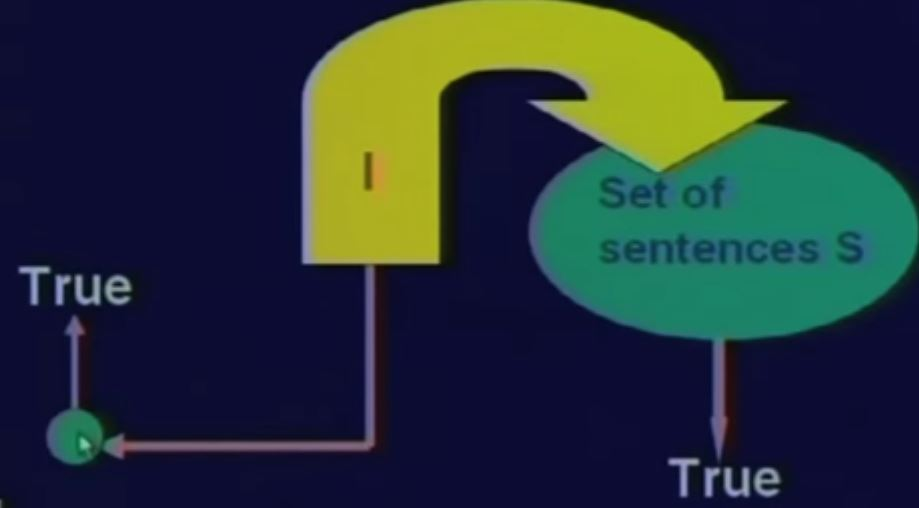# New Technology

Trending Technology Machine Learning, Artificial Intelligent, Block Chain, IoT, DevOps, Data Science## Search This Blog

It rains in July
Rains (July)
ㄱ Rains (November) etc.

If it rains today and one does not carry umbrella he will be drenched
Rains (Today) Λ ᆨ Carry (Umbrella) ⟶ Get_Drenched

Rains (Today) Λ ᆨ Carry (Umbrella, Tom) ⟶ Get_Drenched (Tom)

• Infer the truth value of a proposition logic.
• Reason towards new facts , given a set of propositions
• Prove a proposition give a set of propositional facts
Procedure to deriveDe Morgan's Theorem

ᄀ(PΛQ) = ㄱ PV ㄱQ

ᄀ(PVQ) = ㄱ PV ㄱQRetry the Earlier Quiz

If P is true and Q is true, then are the following true or false ?
1. P➝ Q

Reasoning

P : It is the month of July
Q : It rains
R : P ➝ Q
If it is the month of July then it rains
It is the month of July

Conclude : It rains

P ➝ Q can be written as ᆨ P V Q
Conjoined with P we can write

P Λ ㄱP ∨ Q = (P∨ㄱP ) ⋁ Q

= F ⋁ Q
= Q

Thus irrespective of meaning Modus Ponens allows us to infer the truth of Q

Modus Ponens is an inference rule that allows us to deduce the truth of a consequent depending on the truth of the antecedents

Inference rules can be mechanically applied
Other rules
If P and Q then P
If P then P or Q
If Not (Not(P) then P
Chain rule:
If P then Q                                       If Q then R
leads to If P then R

Satisfiability

Remember : An interpretation is a mapping to a world

A sentence is SATISFIABLE  by an interpretation

if
under that interpretation the sentence evaluates to True

If No interpretation makes all the sentences in the set to be true

Then the set of sentences is UNSATISFIABLE or INCONSISTENT

EntailmentIf a sentence s1 has a value True for all interpretations that make all sentences a set of sentence S then

S  ㅏs1
s1 logically follows S
s1 is a logical consequence of S
S logically entails s1Clause : A Special Form

Literal -- A single proposition or its negation  P, ㄱP

A clause is a disjunction of literals P∨ Q∨ ㄱR

Resolution - A technique of Inference
A sound inference mechanism

Principle :
Suppose x is a literal and S1 and S2 are two sets of propositional sentences represented n clausal form

If we have (x V S1) AND (ㄱX V S2)
Then we get S1 V S2
Here S1 V S2 is the resolvent,
x is resolved upon

An Example
• If a triangle is equilateral then it is isosceles
• If a triangle is isosceles then two sides AB and AC are equal
• If AB and AC are equal then angle B and angle C are equal
• ABC is an equilateral triangle
• Angle B is equal to angle C -- Prove